Function Repository Resource:

# GeometricProgression

Produce a set of values in geometric progression

Contributed by: Pedro Cabral
 ResourceFunction["GeometricProgression"][n,r] produces a geometric progression of n terms with ratio r starting at 1. ResourceFunction["GeometricProgression"][n,r,a] produces a geometric progression starting at a.

## Examples

### Basic Examples (3)

Compute the first 10 terms of a geometric progression with ratio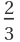:

 In:=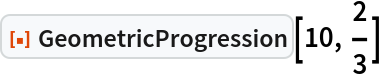Out=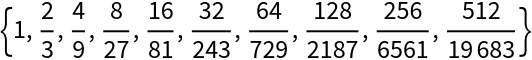Create a ListLinePlot of different geometric progressions:

 In:=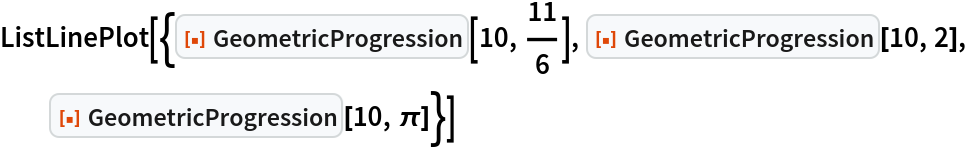Out=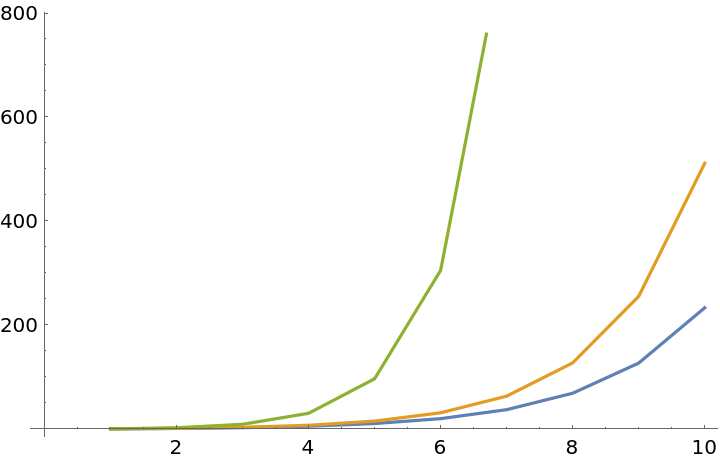Get a geometric sequence of 30 terms with ratio 2, starting at 20:

 In:=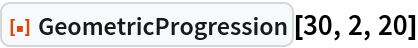Out=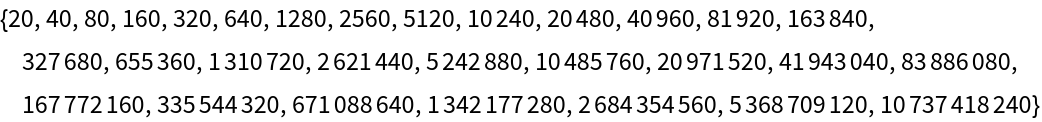### Scope (2)

GeometricProgression can handle complex values:

 In:=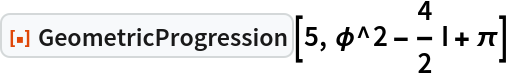Out=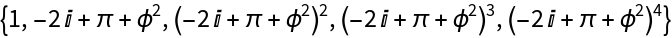GeometricProgression can handle symbols and constants:

 In:=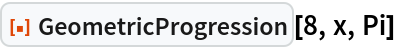Out=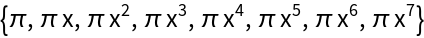Pedro Cabral

## Version History

• 1.0.0 – 09 September 2020